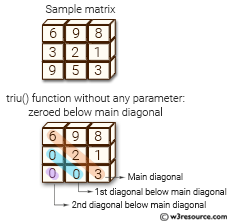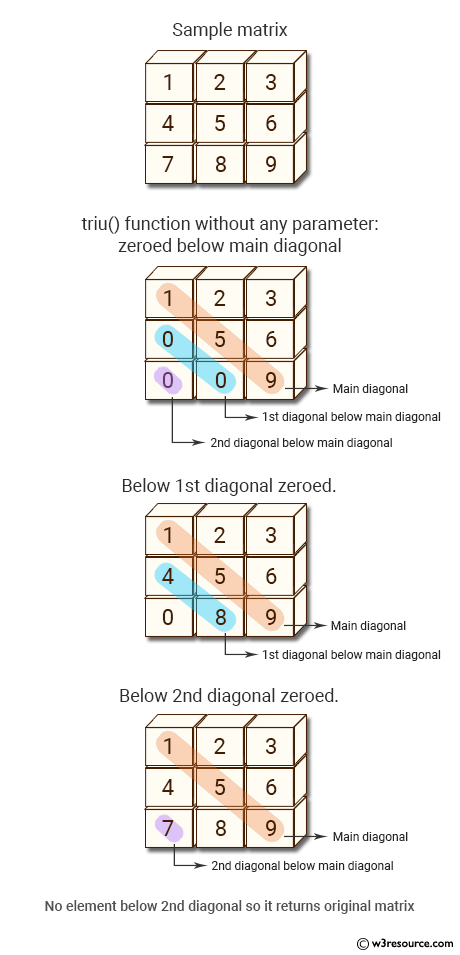# NumPy array creation: triu() function

## numpy.triu() function

Upper triangle of an array.
The triu() function is used to get a copy of a matrix with the elements below the k-th diagonal zeroed.

Syntax:

`numpy.triu(m, k=0)`Version: 1.15.0

Parameter:

Name Description Required /
Optional
m Number of rows in the array.
k Diagonal above which to zero elements. k = 0 (the default) is the main diagonal, k < 0 is below it and k > 0 is above. optional

Return value:

Return a copy of a matrix with the elements below the k-th diagonal zeroed.

Example-1: NumPy.triu() method

``````import numpy as np
m = np.matrix([[1,2,3],[4,5,6],[7,8,9]])
print("Sample matrix")
print(m)
print("\ntriu() function without any parameter:")
print(np.triu(m))
print("\nBelow 1st diagonal zeroed.")
print(np.triu(m,-1))
print("\nBelow 2nd diagonal zeroed.")
print(np.triu(m,-2))
``````

Output:

```Sample matrix
[[1 2 3]
[4 5 6]
[7 8 9]]

triu() function without any parameter:
[[1 2 3]
[0 5 6]
[0 0 9]]

Below 1st diagonal zeroed.
[[1 2 3]
[4 5 6]
[0 8 9]]

Below 2nd diagonal zeroed.
[[1 2 3]
[4 5 6]
[7 8 9]]
```

Pictorial Presentation:Python - NumPy Code Editor:

Previous: tril()
Next: vander()

﻿